# The Bitruncated 120-cell

The bitruncated 120-cell, also known as the bitruncated 600-cell, is a beautiful uniform polychoron bounded by 120 truncated icosahedra and 600 truncated tetrahedra, for a total of 720 cells, 4320 faces (2400 hexagons, 720 pentagons, 1200 triangles), 7200 edges, and 3600 vertices.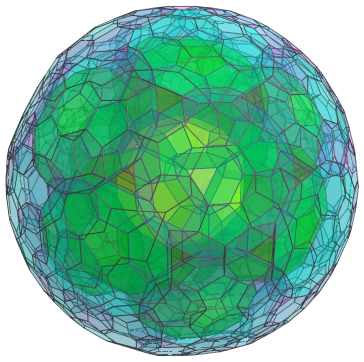The bitruncated 120-cell represents the midpoint in the series of truncations that transforms the 120-cell to the 600-cell, or vice versa. It may be constructed by radially expanding the pentagonal faces of the rectified 120-cell outwards.

## Structure

We shall explore the structure of the bitruncated 120-cell by means of its parallel projection into 3D, centered on one of its truncated icosahedral cells.

### The 120 Truncated Icosahedra

We begin first by looking at the 120 truncated icosahedra, which correspond with the 120 dodecahedra of the 120-cell. The following image shows the closest truncated icosahedron to the 4D viewpoint: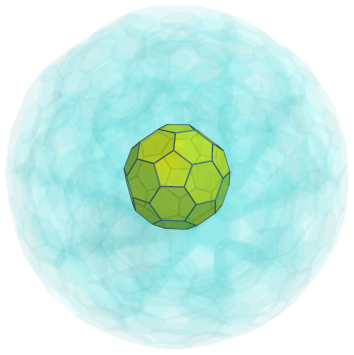To reduce visual clutter, we render all the other cells with a very light transparent color and omit all edges and vertices, except the ones on the nearest cell.

The pentagonal faces of this truncated icosahedron are joined to 12 other truncated icosahedra, shown next: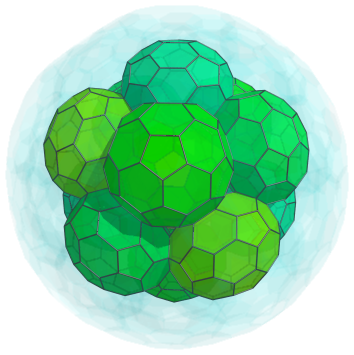Another 20 truncated icosahedra are joined to each triplet of truncated icosahedra above. These are shown next: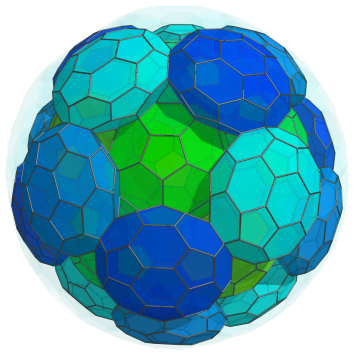These cells appear slightly flattened because they are seen at an angle from the 4D viewpoint. Another 12 truncated icosahedra fit into the visible gaps in the above image, shown below: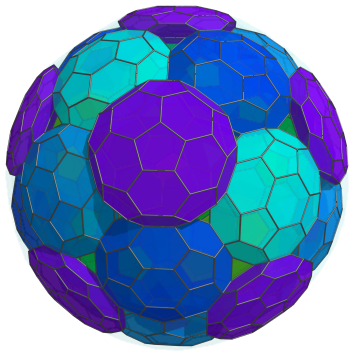All of these cells lie on the “northern hemisphere” of the bitruncated 120-cell. Now we have reached the equator, where there are 30 more truncated icosahedra, as shown below: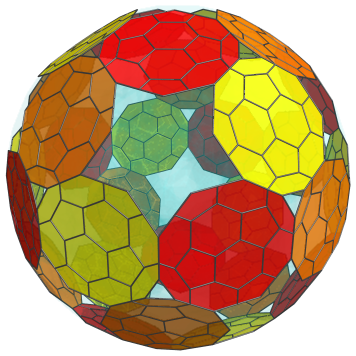For clarity, we omit the cells we've seen previously. These cells have been flattened into decagons, because they lie at a 90° angle to the 4D viewpoint. In 4D they are perfectly uniform truncated icosahedra.

Past this point, the cells in the northern hemisphere repeat themselves in reverse order to form the cells in the southern hemisphere. The pentagrammic gaps in the image of the equatorial cells above correspond with truncated tetrahedral cells, which we omitted here, and the pentagonal faces which the last 12 cells in the northern hemisphere share with the corresponding cells in the southern hemisphere.

In summary, we have 1+12+20+12=45 cells in the northern hemisphere, 30 cells on the equator, and another 45 cells in the southern hemisphere, for a total of 120 truncated icosahedral cells.

### The 600 Truncated Tetrahedra

Now we shall look at the layout of the truncated tetrahedra in the bitruncated 120-cell.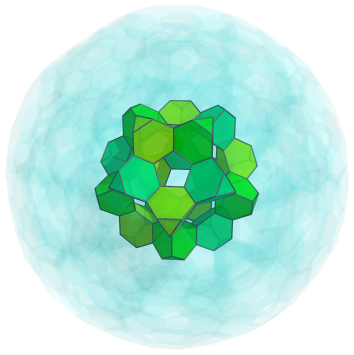The above image shows the first layer of 20 truncated tetrahedra, corresponding with the vertices of a dodecahedron. The void they surround is the truncated icosahedron closest to the 4D viewpoint, shown earlier, which we omit here. They each share a hexagonal face with this truncated icosahedron. These truncated tetrahedra are joined to each other via their triangular faces.

The exposed triangular faces of these truncated tetrahedra are joined to another layer of 20 truncated tetrahedra, corresponding with the vertices of a larger dodecahedron: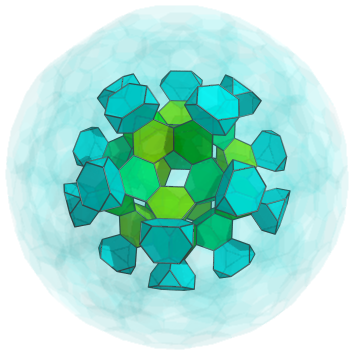These truncated tetrahedra are bridged to each other by 30 other truncated tetrahedra, corresponding with the edges of a dodecahedron: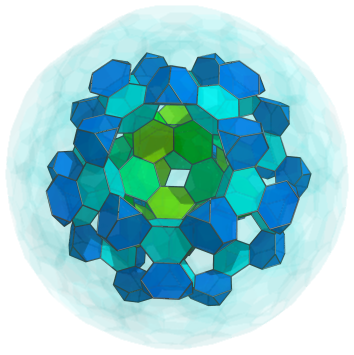Joined to the triangular faces of these 30 truncated tetrahedra are 12 circles of 5 truncated tetrahedra each, making a total of 60 more truncated tetrahedra, as shown below: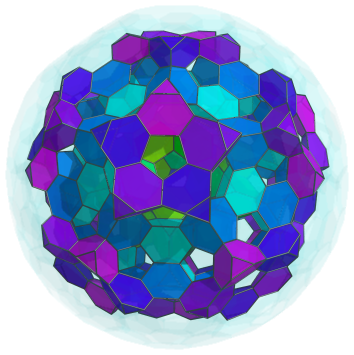The exposed triangular faces of these 60 truncated tetrahedra are joined to another layer of 60 truncated tetrahedra, this time occurring in pairs: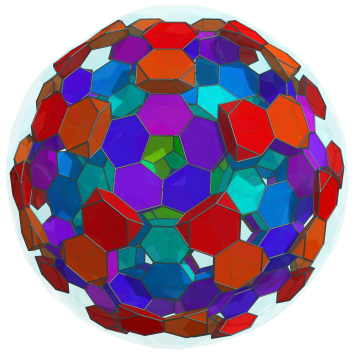These pairs are bridged to each other by another 60 truncated tetrahedra, shown next: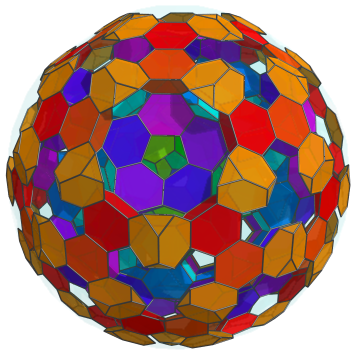Finally, there are another 20 truncated tetrahedra shared by each triplet of these truncated tetrahedra, before we reach the equator. These are shown in yellow below: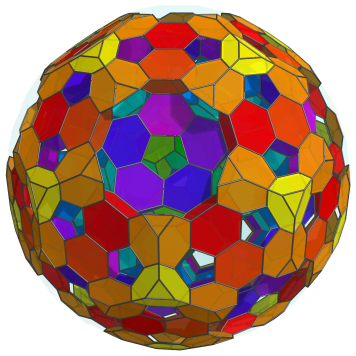Finally, at the equator are 60 truncated tetrahedra in 12 circles of 5 truncated tetrahedra each. These are shown below: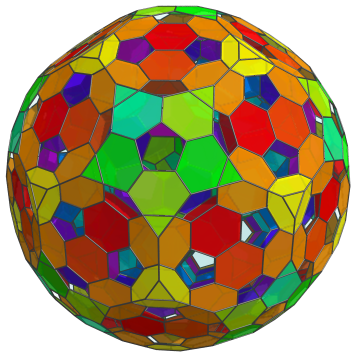These equatorial truncated tetrahedra have been foreshortened into pentagons because they are seen at a 90° angle. They are perfectly uniform in 4D. The next image shows these truncated tetrahedra with the other cells culled, for clarity: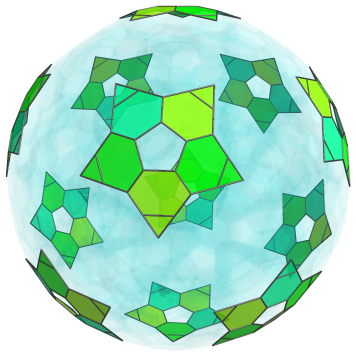And here are all the equatorial cells, including the truncated icosahedra, together: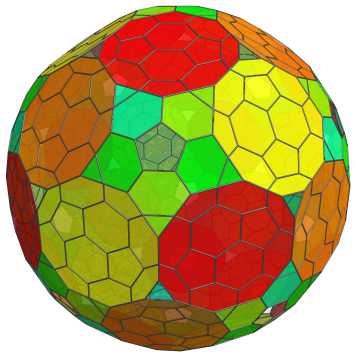The pentagonal gaps, of course, are not images of any equatorial cell, but are where 12 northern hemisphere truncated icosahedra touch their southern hemisphere counterparts.

Past this point, the layout of cells in the northern hemisphere repeats itself in reverse order to form the cells in the southern hemisphere. Therefore, we have 20+20+30+60+60+60+20=270 truncated tetrahedra in the northern hemisphere, 60 truncated tetrahedra on the equator, and 270 truncated tetrahedra in the southern hemisphere, for a total of 600 truncated tetrahedra.

## Coordinates

The Cartesian coordinates of the bitruncated 120-cell, centered on the origin and having edge length 2, are all permutations of coordinates and changes of sign of:

• (0, 0, 4φ, 2φ4)
• (2+φ, 2+φ, 2+5φ, 2+5φ)

along with the even permutations of coordinates and all changes of sign of:

 (0, 1, 4+5φ, 1+5φ) (0, 1, 3φ, 3+7φ) (0, φ, 5φ2, 1+4φ) (0, 3φ2, 3+4φ, 4+3φ) (1, 2φ, 3+7φ, 2+φ) (1, 2φ, 5φ2, φ4) (1, 2, φ5, 2+5φ) (1, 2φ2, φ5, 4+3φ) (φ, 2, φ3, 3+7φ) (φ, φ4, 2+5φ, 4+3φ) (2φ, 1+4φ, 2+5φ, 3φ2) (2φ, φ4, 1+5φ, 3+4φ) (2, 2φ, 2φ4, 2φ2) (2, φ3, 4+5φ, 3φ2) (2, 2+φ, φ5, 3+4φ) (φ3, 4φ, φ5, φ4) (φ3, 2φ2, 1+5φ, 2+5φ) (3φ, 2φ2, 1+4φ, φ5) (2+φ, φ3, 5φ2, 2φ2) (2+φ, 3φ, 4+5φ, φ4)

where φ=(1+√5)/2 is the Golden Ratio.

Last updated 18 Jun 2019.